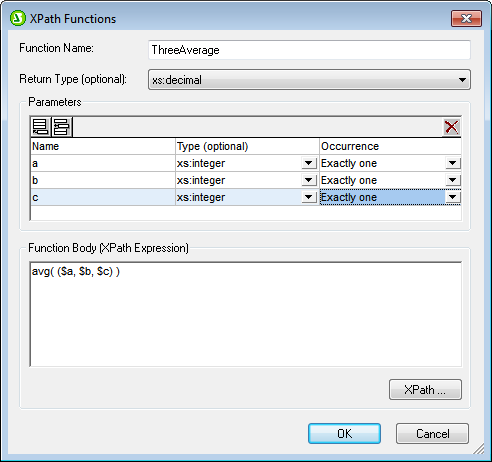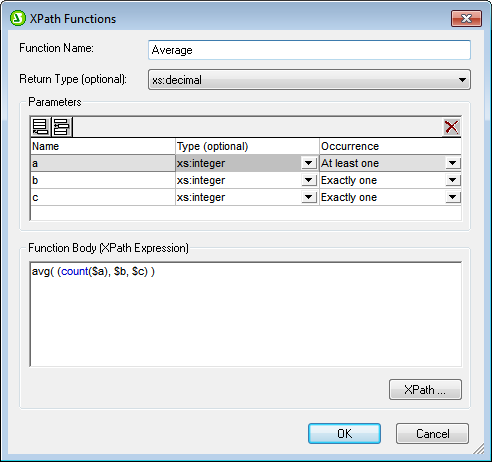# Altova StyleVision 2020 Basic Edition

 Parameters and Sequences Scroll Home Prev Top Next More

It is important to note the relationship between parameters and sequences, and how parameters and sequences are used in XPath expressions. We use the following definitions to make these relationships clearer:

A sequence consists of items that are either atomic values or nodes. A comma can be used to construct a sequence, by placing it between the items of a sequence and so allowing the sequence to be built.

An XPath function can be defined to take parameters. For example, in the XPath 2.0 expression count(\$a), the part within the function's parentheses is the parameter of the function and it must be a sequence of items.

An argument consists of one or more items in a function call. For example, the function count(//Person) has one argument: //Person. This argument is valid because it returns one sequence of nodes, which corresponds to the signature of the count() function. (The signature of a function specifies the number of parameters and the expected datatype of each parameter. It also specifies what the function will return and the datatype of the returned object)

The function substring('StyleVisionExamples', 6, 6)which returns the string Visionhas three arguments. This is valid according to the signature of the substring() function, and is specified by it. When a function call has multiple arguments, these are separated by commas.

## Parentheses as sequence delimiters

A key point to note when constructing XPath expressions is this: Parentheses are used to delimit sequences that use the comma separator or range operator to enumerate sequences. As a result, each parentheses-delimited sequence is read as one parameter (in function definitions) or one argument (in function calls).

Parentheses are not necessary around a path (or locator) expression (example of a path expression: //Person/@salary), because a path expression can be read unambiguously as one parameter or one argument. It is in fact a one-sequence parameter/argument.

Here are some examples to illustrate the points made above:

avg((10, 20, 30)) The avg function of XPath 2.0 takes one sequence of items as its  single argument. Since this sequence is a comma-separated enumeration, the inner pair of parentheses are necessary in order to delimit the mandatory single sequence. Without the inner parentheses, the argument would have three arguments and therefore be invalid. (The outer pair of parentheses are the parentheses of the function.)

avg(//Person/@salary) This path expression selects the salary attribute nodes of all Person elements and returns their attribute-values as the sequence to be evaluated (that is, to be averaged). No parentheses are required because the sequence is not enumerated before the argument is read. The argument is the single path (or locator) expression. The path expression is evaluated and the returned values are submitted to the function as the items of a sequence.

count((10 to 34)) This is an enumeration via the range operator. The range operator 'to' generates  a sequence of comma-separated items (the integers from 10 to 34) before the argument is read. As a result, the count() function has within its argument a comma-separated sequence of 25 items. To read this as one single-sequence argument, delimiting parentheses are required. Without such parentheses, the function call would have 25 arguments instead of onethus invalidating the function call, since the count() function must, according to its signature, have only one argument.

count((10 to 34, 37)) The inner parentheses indicate that everything within them is the one argument of the function calla single sequence consisting of 26 items.

count(//Person) No sequence-delimiter parentheses are required around the single argument. The arguent is a path expression that collects the //Person nodes in the XML document and returns these nodes as the items of the sequence to be counted.

## Using XPath parameters in XPath functions

When parameters are used in the definition of a user-defined XPath function, ensure (i) that the number of arguments in a function call to this user-defined XPath function is correct, and (ii) that the arguments evaluate correctly to the expected type and occurrence.The screenshot above defines three parameters (in the Parameters pane) and then uses these parameters (in the Function Body pane) to define an XPath function.

Each parameter that is defined in the Parameters can be considered to be a single sequence. The number of items allowed within the single sequence is specified with the Occurrence property. In the definition above, each parameter is defined (in its Occurrence property) as a singleton-sequence (that is, a sequence of exactly one item). Each argument of the function call must therefore be a sequence of one item. The Type property specifies the datatype of the items of the sequence.

In the definition of our example XPath function (in the Function Body pane), each parameter provides one item of the sequence that is to be averaged. Since the XPath parameters together constitute a sequence, the sequence must be enclosed in parentheses to ensure that the entire sequence is read as the one parameter of the avg() function. If, at runtime, any of the arguments in the function call (corresponding to the three parameters) is not a singleton-sequence, an error is returned.

Given below are examples of XPath parameter usage in calls to the XPath function ThreeAverage() shown in the screenshot above. In Design View, you can insert an Auto-Calculation and give it the XPath expressions listed below to see the results. The function has been defined to take a sequence of three integers and average them.

sps:ThreeAverage(10,20,30) returns 20. There are three valid arguments in the function call, corresponding to the three XPath parameters.

sps:ThreeAverage( (10),(20),(30) ) returns 20. There are three valid input arguments, corresponding to the three XPath parameters. Each input argument has been enclosed with parentheses (which are redundant, since each sequence is a singleton-sequence; however, this redundancy is not an error).

sps:ThreeAverage( (10),20,30 ) returns 20. There are three valid input arguments, corresponding to the three XPath parameters. The first argument has been enclosed with parentheses (redundant, but not an error).

sps:ThreeAverage( (10,20),(30),(40) ) returns an error because the first argument is not valid. It is not a singleton-sequence, as required by the property definition of the first \$a parameter ('Exactly one').

sps:ThreeAverage( (10,20,30) ) returns an error because only one input argument is submitted, inside the parentheses. Additionally, the argument is invalid because the sequence is not a singleton-sequence.

If the Occurrence property of a parameter is set to At-least-one (as in the definition shown in the screenshot below), then that parameter is defined as a sequence of one-or-more items.In the definition above, the first parameter has been defined as a sequence of one or more items, the next two parameters as singleton-sequences. The function has been defined to count the number of items submitted by the first parameter, add the result to the sum of the two integers submitted by the other two parameters, and then divide the result by three to obtain the average. Notice the following:

The sequence that is the parameter of the avg() function is enclosed in parentheses. This is to specify that the avg() function takes a single sequence consisting of three items as its parameter. The single sequence consists of three integers: the first submitted by the count() function; the second and third are the two parameters b and c.

The argument of the count() function is not enclosed in sequence-delimiter parentheses because the argument is unambiguously a single sequence.

Here are examples of parameter usage in calls to the XPath function Average() shown in the screenshot above.

sps:Average((1,2),3,4) returns 3. There are three valid input arguments, corresponding to the three parameters. The first argument is enclosed in parentheses to delimit it. When the count() function operates on it, the function will return the value 2, which will be the first item of the sequence submitted to the avg() function.

sps:Average( 4,4,4 ) returns 3. There are three valid input arguments. The first argument is allowed to be a sequence of one item (see the Occurrence property of its corresponding parameter). No parentheses are required to indicate separate arguments.

The following additional points should be noted:

If an parameter is defined as having At-least-one occurrence, then a function such as MyAverage() could be defined with an XPath expression such as avg((\$a)). This function would accept an argument that is a single sequence of one-or-more items. The function could be called as follows: sps:MyAverage((2,3,4)), and it would return the value 3. The input argument must be enclosed in parentheses to ensure that the input is being read as a single sequence rather than as three singleton-sequences (which would be the case if there were no enclosing parentheses).

If an XPath parameter \$a is defined as having None-or-one occurrence, then a function such as MyAverage() could be defined with an XPath expression such as avg((\$a, \$b, \$c)). This function would accept as its argument three sequences, with the possibility of the first sequence being empty. If the first sequence is to be empty, then an empty sequence must be explicitly submitted as the first input argument. Otherwise an error is reported. If the function were called as follows: sps:MyAverage(30,20,10), it would return the value 20. The function could also be called with: sps:MyAverage((),20,10), returning 15 (note that the empty sequence does count: as an input value of empty; for a return value of 10, the first item would have to be 0). The following, however, would generate an error: sps:MyAverage(20,10), because no first empty sequence is supplied and, as a consequence, the third input argument is considered to be absent.

## Complex examples

Besides providing the benefit of being able to re-use an XPath expression, user-defined XPath functions also enable the construction of complex customized XPath functions that are not available in the XPath 2.0 function set. For example, a factorial function could easily be constructed with an XPath expression that takes a singleton-sequence as its single parameter. If the parameter \$num is the number to be factorialized, then the XPath expression to create the function would be:

if (\$num < 2) then 1 else \$num * sps:Factorial(\$num - 1)

If this function were called Factorial(), then the factorial of, say 6, could be obtained by calling the function with: sps:Factorial(6).